Home > GC > Chapter 6 > Lesson 6.2.4 > Problem6-72

6-72.
1. the geometric relationships in each of the diagrams below. For each one, write and solve an equation to find the value of the variable. Name all geometric relationships or conjectures that you use. Homework Help ✎

1.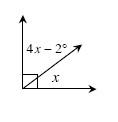2.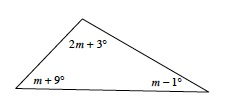3.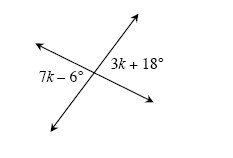4.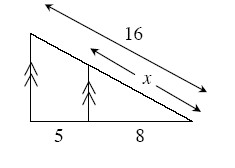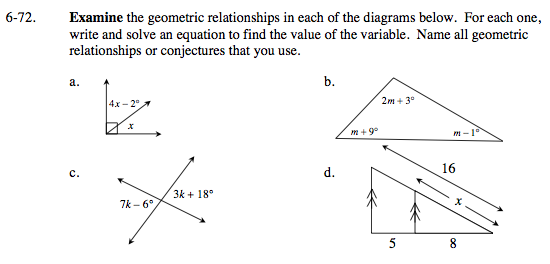What type of angle do these two angles sum to?

x = 18.4

How do these angles relate to each other?

What do all the angles of a triangle sum to?

m = 42.25

These triangles are similar by AA~.
Since the triangles are similar, the ratios of corresponding sides are equal.

x = 9.8